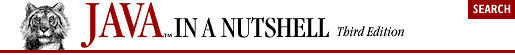## 4.3. Numbers and Math

Java provides the byte, short, int, long, float, and double primitive types for representing numbers. The java.lang package includes the corresponding Byte, Short, Integer, Long, Float, and Double classes, each of which is a subclass of Number. These classes can be useful as object wrappers around their primitive types, and they also define some useful constants:

```// Integral range constants: Integer, Long, and Character also define these
Byte.MIN_VALUE      // The smallest (most negative) byte value
Byte.MAX_VALUE      // The largest byte value
Short.MIN_VALUE     // The most negative short value
Short.MAX_VALUE     // The largest short value

// Floating-point range constants: Double also defines these
Float.MIN_VALUE     // Smallest (closest to zero) positive float value
Float.MAX_VALUE     // Largest positive float value

// Other useful constants
Math.PI             // 3.14159265358979323846
Math.E              // 2.7182818284590452354```

A Java program that operates on numbers must get its input values from somewhere. Often, such a program reads a textual representation of a number and must convert it to a numeric representation. The various Number subclasses define useful conversion methods:

```String s = "-42";
byte b = Byte.parseByte(s);             // s as a byte
short sh = Short.parseShort(sh);        // s as a short
int i = Integer.parseInt(s);            // s as an int
long l = Long.parseLong(s);             // s as a long
float f = Float.parseFloat(s);          // s as a float (Java 1.2 and later)
f = Float.valueOf(s).floatValue();      // s as a float (prior to Java 1.2)
double d = Double.parseDouble(s);       // s as a double (Java 1.2 and later)
d = Double.valueOf(s).doubleValue();    // s as a double (prior to Java 1.2)

// The integer conversion routines handle numbers in other bases
byte b = Byte.parseByte("1011", 2);      // 1011 in binary is 11 in decimal
short sh = Short.parseShort("ff", 16);   // ff in base 16 is 255 in decimal

// The valueOf() method can handle arbitrary bases
int i = Integer.valueOf("egg", 17).intValue();   // Base 17!

// The decode() method handles octal, decimal, or hexadecimal, depending
// on the numeric prefix of the string
short sh = Short.decode("0377").byteValue();   // Leading 0 means base 8
int i = Integer.decode("0xff").shortValue();   // Leading 0x means base 16
long l = Long.decode("255").intValue();        // Other numbers mean base 10

// Integer class can convert numbers to strings
String decimal = Integer.toString(42);
String binary = Integer.toBinaryString(42);
String octal = Integer.toOctalString(42);
String hex = Integer.toHexString(42);
String base36 = Integer.toString(42, 36);```

Numeric values are often printed differently in different countries. For example, many European languages use a comma to separate the integral part of a floating-point value from the fractional part (instead of a decimal point). Formatting differences can diverge even further when displaying numbers that represent monetary values. When converting numbers to strings for display, therefore, it is best to use the java.text.NumberFormat class to perform the conversion in a locale-specific way:

```import java.text.*;

// Use NumberFormat to format and parse numbers for the current locale
NumberFormat nf = NumberFormat.getNumberInstance();  // Get a NumberFormat
System.out.println(nf.format(9876543.21)); // Format number for current locale
try {
Number n = nf.parse("1.234.567,89");     // Parse strings according to locale
} catch (ParseException e) { /* Handle exception */ }

// Monetary values are sometimes formatted differently than other numbers
NumberFormat moneyFmt = NumberFormat.getCurrencyInstance();
System.out.println(moneyFmt.format(1234.56)); // Prints \$1,234.56 in U.S. ```

The Math class defines a number of methods that provide trigonometric, logarithmic, exponential, and rounding operations, among others. This class is primarily useful with floating-point values. For the trigonometric functions, angles are expressed in radians. The logarithm and exponentiation functions are base e, not base 10. Here are some examples:

```double d = Math.toRadians(27);       // Convert 27 degrees to radians
d = Math.cos(d);                     // Take the cosine
d = Math.sqrt(d);                    // Take the square root
d = Math.log(d);                     // Take the natural logarithm
d = Math.exp(d);                     // Do the inverse: e to the power d
d = Math.pow(10, d);                 // Raise 10 to this power
d = Math.atan(d);                    // Compute the arc tangent
d = Math.toDegrees(d);               // Convert back to degrees
double up = Math.ceil(d);            // Round to ceiling
double down = Math.floor(d);         // Round to floor
long nearest = Math.round(d);        // Round to nearest```

The Math class also defines a rudimentary method for generating pseudo-random numbers, but the java.util.Random class is more flexible. If you need very random pseudo-random numbers, you can use the java.security.SecureRandom class:

```// A simple random number
double r = Math.random();     // Returns d such that: 0.0 <= d < 1.0

// Create a new Random object, seeding with the current time
java.util.Random generator = new java.util.Random(System.currentTimeMillis());
double d = generator.nextDouble();   // 0.0 <= d < 1.0
float f = generator.nextFloat();     // 0.0 <= d < 1.0
long l = generator.nextLong();       // Chosen from the entire range of long
int i = generator.nextInt();         // Chosen from the entire range of int
i = generator.nextInt(limit);        // 0 <= i < limit (Java 1.2 and later)
boolean b = generator.nextBoolean(); // true or false (Java 1.2 and later)
d = generator.nextGaussian();        // Mean value: 0.0; std. deviation: 1.0
byte[] randomBytes = new byte;
generator.nextBytes(randomBytes);    // Fill in array with random bytes

// For cryptographic strength random numbers, use the SecureRandom subclass
java.security.SecureRandom generator2 = new java.security.SecureRandom();
// Have the generator generate its own 16-byte seed; takes a *long* time
generator2.setSeed(generator2.generateSeed(16));  // Extra random 16-byte seed
// Then use SecureRandom like any other Random object
generator2.nextBytes(randomBytes);   // Generate more random bytes```

The java.math package contains the BigInteger and BigDecimal classes. These classes allow you to work with arbitrary-size and arbitrary-precision integers and floating-point values. For example:

```import java.math.*;

// Compute the factorial of 1000
BigInteger total = BigInteger.valueOf(1);
for(int i = 2; i <= 1000; i++)
total = total.multiply(BigInteger.valueOf(i));
System.out.println(total.toString());```Copyright © 2001 O'Reilly & Associates. All rights reserved.